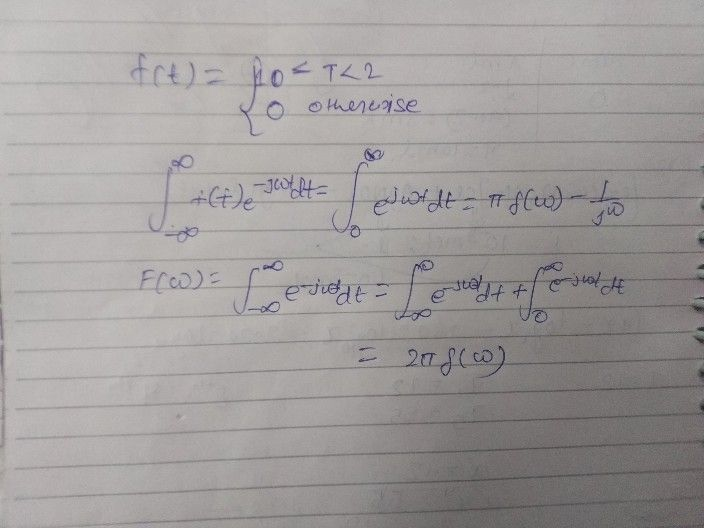Symbol
ProblemProblem $2$ Find the Fourier transform for the following functions, a) $\right)f\left(t\right)= \begin{cases} 1 \\ 0 \end{cases}$ $0<t<2$ otherwise $\left(10$ $Man^{1}<s\right)$ b) $\right)f\left(t-5\right)$ if $F\left(f\left(t\right)\right)=\dfrac {1} {\sqrt{2\pi } }sinc\left(w\right)$